## Unity游戏中使用贝塞尔曲线_u010019717的博客-程序员宝宝_贝塞尔曲线游戏app

贝塞尔曲线是最基本的曲线，一般用在计算机 图形学和 图像处理。贝塞尔曲线可以用来创建平滑的曲线的道路、 弯曲的路径就像 祖玛游戏、 弯曲型的河流等。

一条贝塞尔曲线是由一组定义的控制点 P0到 Pn，在 n 调用它的顺序 (n = 1 为线性，2 为二次，等.)。第一个和最后一个控制点总是具有终结点的曲线;然而，中间两个控制点 (如果有的话) 一般不会位于曲线上 。

P = P0+ t (P1 - P0)，0 <= t <= 1

For T = 0，P = P0.

For T = 1，P = P1.

For T = 0.5，P =  P0和 P1间的点.

B(t) = P0+ t (P1 -  P0) = (1-t) P0 + tP1    ,0 <= t <= 1

线性贝塞尔曲线如何计算出来的是如下所示: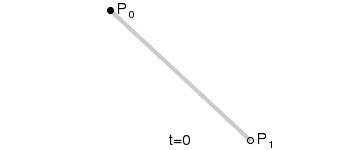二次贝塞尔曲线具有三个控制点。二次贝塞尔曲线是点对点的两个线性贝塞尔曲线的线性插值。为给出了三个点 P0、P1和 P2一条二次贝塞尔曲线，其实是两条线性的贝塞尔曲线，线性贝塞尔曲线的 P0和 P1和   线性贝塞尔曲线P1和 P2.     所以，给出二次贝塞尔曲线 ：

B(t) = (1-t) BP0P1(t) + t BP1P2(t)，0 <= t <= 1

B(t) = (1-t) [(1-t) P0 + tP1] + t [(1-t) P1+ tP2]，0 <= t <= 1

B(t) = (1-t)2P0+ 2 (1-t) tP1 + t2P2,   0 <= t <= 1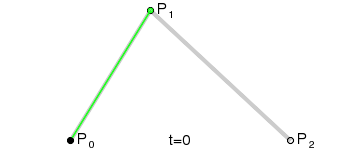B(t) = (1-t) BP0,P1,P2(t) + t BP1,P2,P3(t)，0 <= t <= 1

B(t) = (1-t) [(1-t)2P0+ 2 (1-t) tP1 + t2P2] + t [(1-t)2P1+ 2 (1-t) tP2 + t2P3]，0 <= t <= 1

B(t) = (1-t)3P0 + 3(1-t)2tP1+ 3 (1-t) t2P2 + t3P3           0 <= t <= 1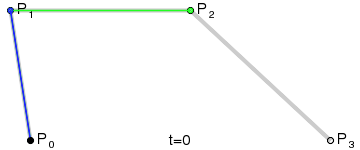在大多数应用程序使用两种二次或三次方贝塞尔函数。然而，你总可以使用更高程度贝塞尔函数绘制更复杂的曲线，但较高程度贝塞尔函数的计算是比较复杂和增加处理开销。所以，而不是使用更高的学位贝塞尔函数绘制更复杂的曲线，你可以多次使用两种二次或三次方贝塞尔函数。在这里，我创建了一个演示和绘制的∞形曲线，使用三次方贝塞尔函数中循环，如下所示。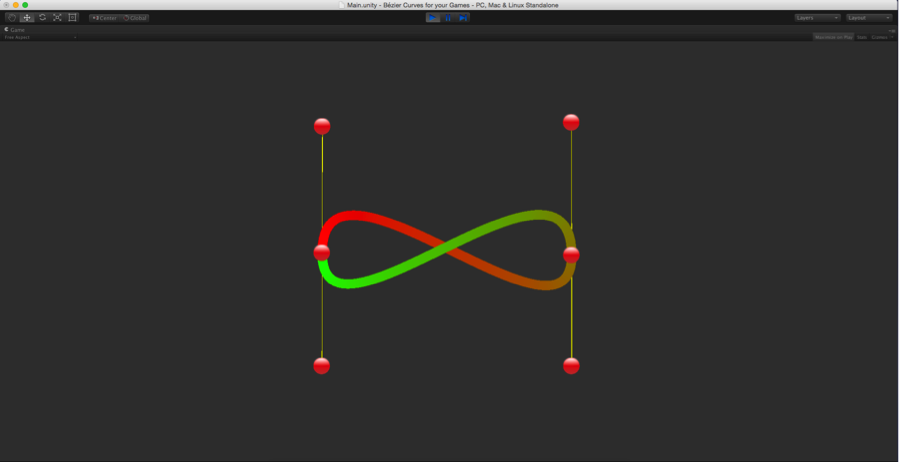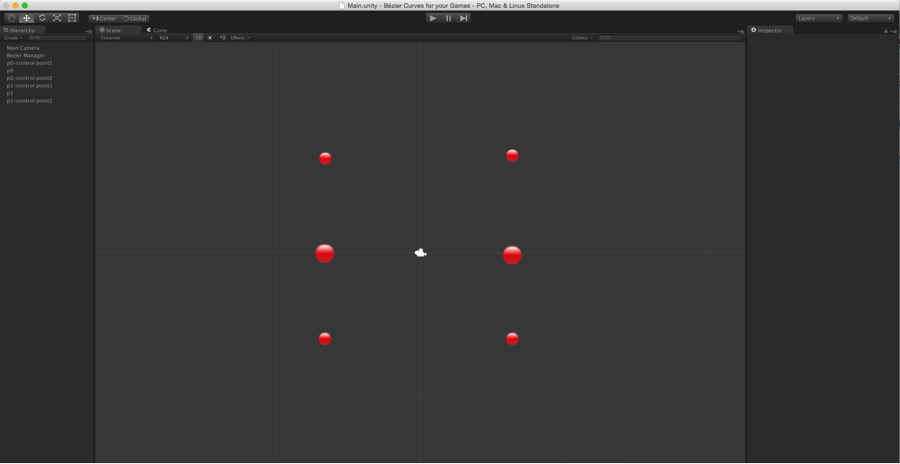Bezier.cs:

``````using UnityEngine;
using System.Collections.Generic;
[RequireComponent(typeof(LineRenderer))]
public class Bezier : MonoBehaviour
{
public Transform[] controlPoints;
public LineRenderer lineRenderer;

private int curveCount = 0;
private int layerOrder = 0;
private int SEGMENT_COUNT = 50;

void Start()
{
if (!lineRenderer)
{
lineRenderer = GetComponent<LineRenderer>();
}
lineRenderer.sortingLayerID = layerOrder;
curveCount = (int)controlPoints.Length / 3;
}

void Update()
{

DrawCurve();

}

void DrawCurve()
{
for (int j = 0; j <curveCount; j++)
{
for (int i = 1; i <= SEGMENT_COUNT; i++)
{
float t = i / (float)SEGMENT_COUNT;
int nodeIndex = j * 3;
Vector3 pixel = CalculateCubicBezierPoint(t, controlPoints [nodeIndex].position, controlPoints [nodeIndex + 1].position, controlPoints [nodeIndex + 2].position, controlPoints [nodeIndex + 3].position);
lineRenderer.SetVertexCount(((j * SEGMENT_COUNT) + i));
lineRenderer.SetPosition((j * SEGMENT_COUNT) + (i - 1), pixel);
}

}
}

Vector3 CalculateCubicBezierPoint(float t, Vector3 p0, Vector3 p1, Vector3 p2, Vector3 p3)
{
float u = 1 - t;
float tt = t * t;
float uu = u * u;
float uuu = uu * u;
float ttt = tt * t;

Vector3 p = uuu * p0;
p += 3 * uu * t * p1;
p += 3 * u * tt * p2;
p += ttt * p3;

return p;
}
}``````

Between P0, P0- control Point1, P1- control Point1 and P1.

Between P1, P1- control Point1, P0- control Point2 and P0.

任何控制点  可处理其相应的曲线的曲率。你可以在任何时间改变曲线，通过拖动任意控制点，如下所示: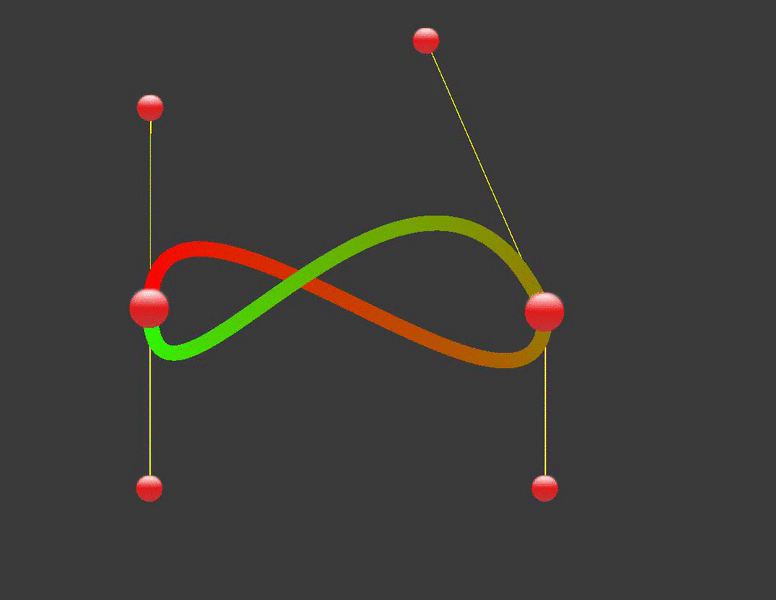### Warning：detected "cgroupfs" as the Docker cgroup driver. The recommended driver is "systemd_xiliangMa的博客-程序员宝宝

Warning：detected “cgroupfs” as the Docker cgroup driver. The recommended driver is "systemd问题描述:kubectl init 或者 kubectl join 出现 Warning：detected "cgroupfs" as the Docker cgroup driver. The recommen...

### ROS URDF语法-实现小车模型构建-URDF工具使用（十六）_啥也不是的py人的博客-程序员宝宝_svga_vgpu10

1.刷新环境变量只是路径下新建了文件，或者有路径相关的变量修改，只是修改文件编码无需source2.source只是写入内存编译，关机后重开要重新编译3.xml文件中注释也是ctrl+/4.在vscode中ctrl+/就是注释5.evince mycar.pdf是打开pdf的命令

### git branch 分支_斜阳雨陌的博客-程序员宝宝_git branch -b

Git自学之路（四）- git branch 分支几乎所有的版本控制系统都以某种形式支持分支。 使用分支意味着你可以把你的工作从开发主线上分离开来，以免影响开发主线。 在很多版本控制系统中，这是一个略微低效的过程——常常需要完全创建一个源代码目录的副本。对于大项目来说，这样的过程会耗费很多时间。有人把 Git 的分支模型称为它的`‘必杀技特性’’，也正因为这一特性，使得 Git 从

### 安装missingno报错_zzh_my的博客-程序员宝宝

missingno可以绘制缺失数据分布图。在安装时报错，信息如下：D:\coding&gt;pip install missingnoDEPRECATION: Python 2.7 will reach the end of its life on January 1st, 2020. Please upgrade your Python as Python 2.7 won't be ...

### powershell静默批量卸载补丁_qq_34860100的博客-程序员宝宝_powershell删除补丁

#17763.2114 补丁系统KB5005030 (OS Build 17763.2114)\$UpdateArray = @（”17763.2114“） foreach (\$UpdateVersion in \$UpdateArray) { \$SearchUpdates = dism /online /get-packages | findstr "Package_for" | findstr "\$UpdateVersion" if (\$SearchUpdate